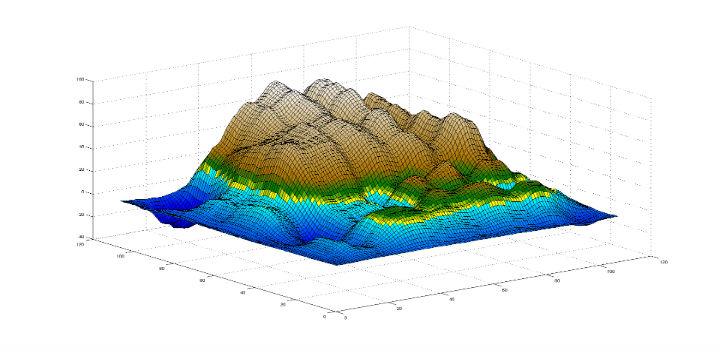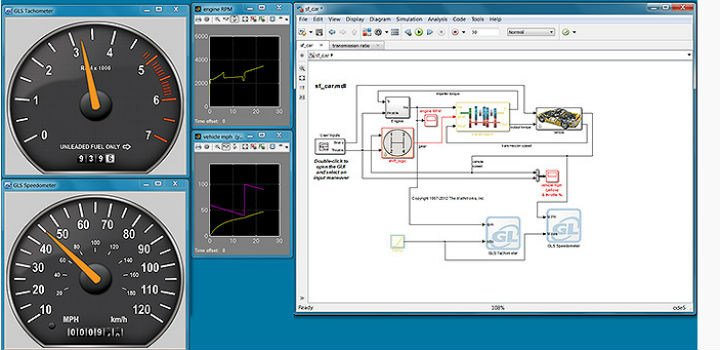# Simulink Projects for Electrical and Electronics Engineering students.

Simulink Projects acts as an interactive graphical programming language library tool. Simulink is used for analyzing, modelling and simulating multi domain dynamic systems. Our concerns carry out simulink projects to enable rapid construction of virtual prototypes at any level of detail with low effort to explore design concepts. Graphical user interface is provided in Simulink Projects for constructing models as block diagrams. Data’s can be absolutely shared among programs when simulink is merged with MATLAB. Block diagramming tools which is a customization set for primary interconnection concepts is used in Simulink Projects.Simulink tool can be scripted in matlab,can also integrated in matlab environment. Simulink tool is developed by mathworks and current stable version as of march 2015 is 8.5 (part of R2015a).

For modeling discrete-time and continuous time system. Libraries of predefined blocks are accessed.
ODE solver in simulation engine with variable step and fixed step
For managing and constructing hierarchical block diagrams in graphical editor.
Legacy code tools are used for importing c or c++ code into models
For managing data and model files, project or data management tools are concentrated.
Data displays and scopes for viewing simulation results• Discrete :Memory, unit delay and function is transferred.
• Sources:It blocks which has only output, constants and generators.
• Sim power system:Measurements, electrical blocks, electrical sources and machines are accompanied in sim power system.
• Sinks:It blocks that has only scope to workspace and input.
• User defined function:S functional builder,S-function.
• Math operation:Sum,Product,Trigonometric functions

• Image or video processing.
• Mechatronics.
• Control system.
• Model based design.
• Digital signal processing.
• Embedded systems.
• Communication systems.

#### Benefits of Carrying Simulink Projects with us ?

• We assure you the quality of simulink projects.
• It is cost effective
• Time to market.
Simulink Projects for EEE Students? Interested to Innovative Simulink Projects? Require Professional Support?
Get Latest IEEE based Matlab simulink projects for EEE students from Industry Experts. Create Original Inventive Simulink Projects.

### Digital modulation techniques using MATLAB simulink Projects.

Three Types of different modulation techniques can be implemented in simulink projects as listed below

• Frequency shift key(FSK).
• Phase shift key(M-PSK).

Modulated signals are produced in ASK, MPSK and FSK by digital communication systems using matlab or simulink software.

• Binary FSK is the simplest FSK.
• A pair of discrete frequencies is transfer binary information.
• 2 carrier waves of FSK -Amplitude of the wave,Message periodic pulse.
• Digital information is transferred through carrier frequency in FSK.
• For modulated signal the output acts as a baseband representation.
• By applying m-array phase shift keying method, the PSK modulates the object.

• For digital modulation techniques. ASK is one of the basics type.
• As comments are represented in green color. We find MATLAB code simple.
• MATLAB assistance is provided in ASK simulation by using simple MATLAB function.A graphical user interface is provided in simulink for constructing models as block diagrams. Our experts construct graphical models of systems for simulink projects. The data’s can be absolutely shared among programs when simulink is merged with MATLAB. Digital communication systems using MATLAB and SIMULINK recent trend advancements are studied by our technicians to benefit academic students, PhD’s and research scholars.

0+